# Question 2 (4 points) In a study of cereal leaf beetle damage in oats, researcher measured...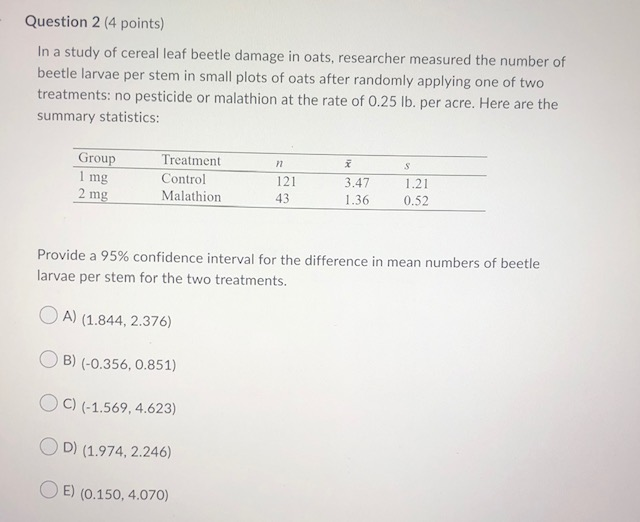Question 2 (4 points) In a study of cereal leaf beetle damage in oats, researcher measured the number of beetle larvae per stem in small plots of oats after randomly applying one of two treatments: no pesticide or malathion at the rate of 0.25 lb. per acre. Here are the summary statistics: n > Group 1 mg S Treatment Control Malathion 2 mg 121 43 3.47 1.36 1.21 0.52 Provide a 95% confidence interval for the difference in mean numbers of beetle larvae per stem for the two treatments. A) (1.844, 2.376) B) (-0.356, 0.851) C) (-1.569, 4.623) OD) (1.974, 2.246) OE) (0.150, 4.070)

Solution :

In a study of cereal leaf beetle in oats, researcher measured the number of beetle larvae per stem in small plot of oats after randomly applying one of two treatments : no pesticides or malathion at the rate of 0.25 lv. Per acre. Here the summary of Statistics is given by,

For Group 1 ( 1 mg ),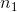= 121.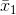= 3.47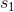= 1.21

For Group 2 ( 2 mg ),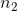= 43,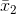= 1.36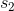= 0.52

TO Find : 95 % confidence interval for the difference in mean.

We know that ,

( 1-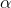) 100% confidence interval is given by,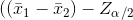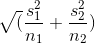,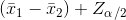)

Now ,

95 % confidence interval for difference in mean is,

(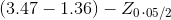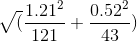,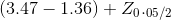)

= (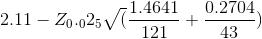,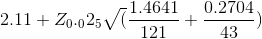)

=(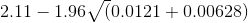,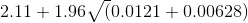). ......(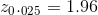by using z table )

= (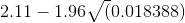,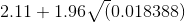)

= (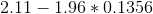,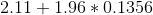)

= (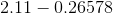, 2.11+ 0.26578 )

= ( 1.8442 , 2.3757 )

i.e. 95 % confidence interval for the difference in mean number of beetle larvae per stem for the two treatments is ( 1.844 , 2.376 )

Answer : Option A is correct ( 1.844, 2.376 )